# Maximal Square

## Introduction to the problem statement

Today we’ll be examining one of the most important questions of dynamic programming, which is often being asked in various product-based companies.

As the title suggests, we need to find the maximal square from the given matrix and return its area. Before getting to the problem statement, let us understand the fundamentals of the square.

So, a square is a shape that has four straight sides of the same length and four angles of 90 degrees (right angles). And for the matrix, a square can be defined as the equal no. of rows and columns.

maximal square is that square that holds the maximum and equal no. of rows and columns in the given matrix.

Now that you have understood the meaning of the question let us have a look at the problem statement:-

Given a binary matrix with 0’s and 1’s, find out the maximum size square sub-matrix with all 1’s and return its area.

Example 1:-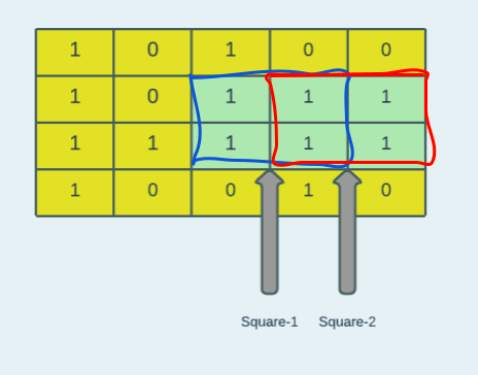Input: matrix = [[1,0,1,0,0],[1,0,1,1,1],[1,1,1,1,1],[1,0,0,1,0]]

Output: 4

Explanation: Since the maximal square is of size 2, output(area) = side x side = 2 x 2 = 4.

Example 2:-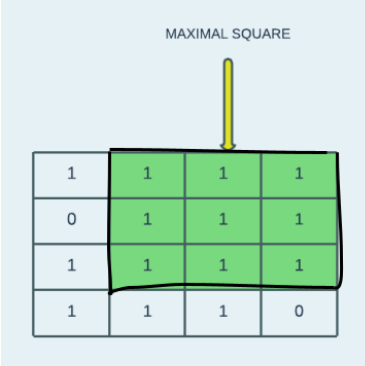Input: matrix = [[1,1,1,1],[0,1,1,1],[1,1,1,1],[1,1,1,0]]

Output: 9

Explanation: Since the maximal square is of size 3, output(area) = side x side = 3 x 3 = 9.

Example 3: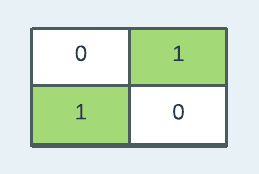Input: matrix = [[0,1],[1,0]]

Output: 1

Explanation: Since the maximal square is of size 1, output(area) = side x side = 1 x 1 = 1.

Example 4:

Input :matrix = [ ]

Output: 0

Explanation: Since the matrix is empty and hence no square is possible, output(area) = 0.

It is recommended to try the stated problem on your own before moving to the solution.

As previously said, the problem we are facing can be handled utilising the dynamic programming technique; thus, before we go into the approach, let us first clarify what dynamic programming is.

## What is Dynamic Programming?The picture above conveys a great deal about Dynamic Programming. So, is it a good idea to keep doing things for which you already have an answer? A coder would beg to differ. That is the essence of Dynamic Programming. Always remember the solutions to sub-problems you've already solved.

To be more specific, A problem must be broken down into a succession of overlapping sub-problems, and solutions to bigger and larger sub-problems must be built up. You've encountered a DP problem if you're given a problem that can be broken down into smaller sub-problems, and these smaller subproblems can be broken down into more smaller ones - and you figure out that there are some overlapping subproblems.

• Dynamic Programming's main notion is to prevent repeating work by remembering partial outcomes, and this concept is used in various real-world scenarios.

• Dynamic Programming is a sophisticated technique that allows you to solve various problems in O(n^2) or O(n^3) time when a naïve approach requires exponential time.

Since now we have gained the idea behind dynamic programming, let us try to think in the same way to solve the given problem.

## Approach

The objective is to traverse the matrix from the last element and go forward until we reach the first element. In addition to traversing, we'll regard each element as the top-left element of a square, assigning the size of the square that can be formed with that element to the newly generated array.

Let us take one example to understand the idea more clearly: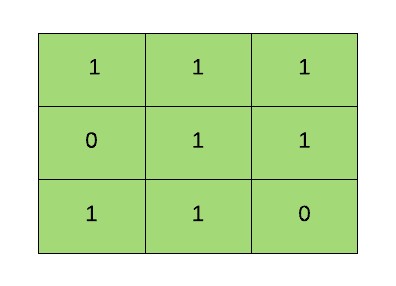Step 1:- Let us take the last element as the top-left element of the square in the above example:

Since it is zero and we are told to consider only 1’s, it won’t be counted, and we can simply assign this element to the other matrix, which has the same size as the given matrix.

Step 2:- Jump to the next element, 1, where we can only build one square with one row and one column.

If we look closely, we can see that any element in the last row or column can create a square of size 1.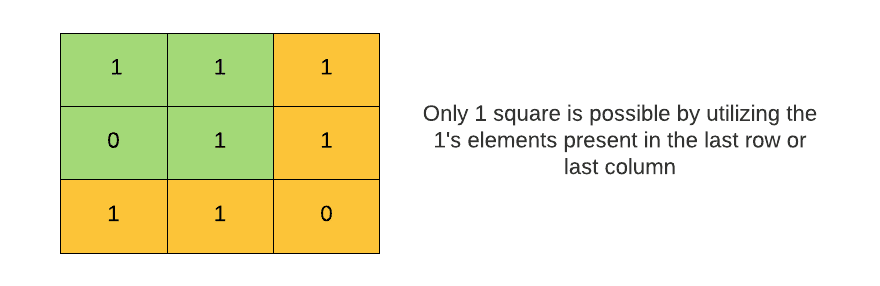Step 3:- Now, let us have a look at the rest portion of the matrix.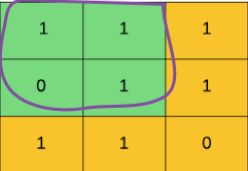In this case, we'll use dynamic programming to access the elements contained in the auxiliary matrix.

The goal is to identify the smallest element in the other matrix from the next element, the vertical element and the diagonal element.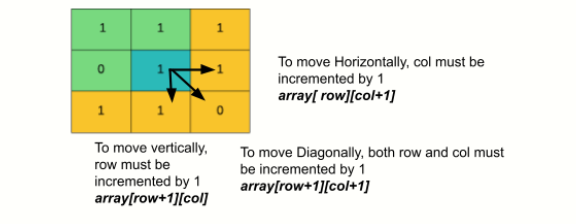The element with the lowest value in the auxiliary matrix will now be increased by one. In this example, the 0 element is the smallest of the 1,1 and 0 elements; consequently, it will be incremented by 1 and then placed in the auxiliary matrix. The result shows that the element we viewed as the top-left of the square will make a square of size 1.

If we proceed, the block contains the 0's element; thus, it will not be tallied and put immediately into the matrix.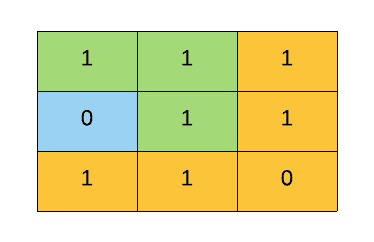We're now at the top right corner of the matrix, and we've agreed that every time we come across an element in the last row or column, we'll just add it to the matrix.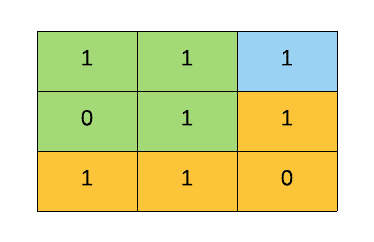So far, our auxiliary matrix has the following elements: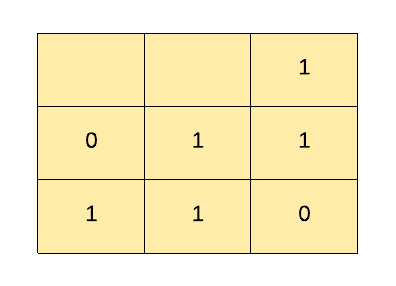Step 4:- Now, we are at the second element, i.e., 1.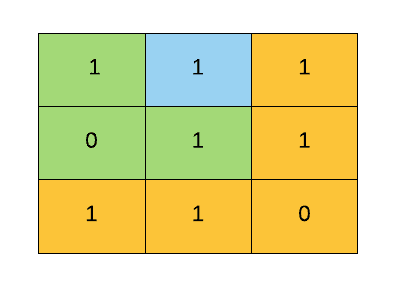The same processes will be taken to determine which element is the smallest.

Please keep in mind that the element comparison must be made in the auxiliary matrix.

Because there are 1s in other blocks of the auxiliary matrix, the minimum element is now 1. It will now be increased by one. The outcome is = 1+1 = 2, which will be saved in the matrix.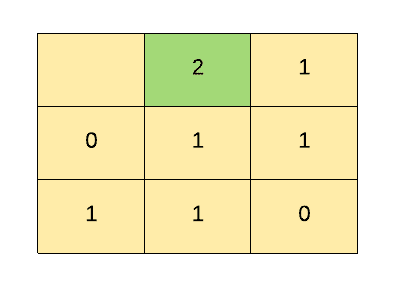If we move further without updating the result in the answer variable, which we used to return the area, the result would be wrong.

Hence, as soon as the element in the other matrix is greater than the element in the answer variable, the answer gets updated.

If we move to the next element, the first element in the matrix, the same process will be repeated by determining the minimum element.

Here we have the options ( 2(horizontal) , 1(diagonal) , 0(bottom)). The minimum element is 0; it is incremented by 1 and stored in the matrix as shown below:-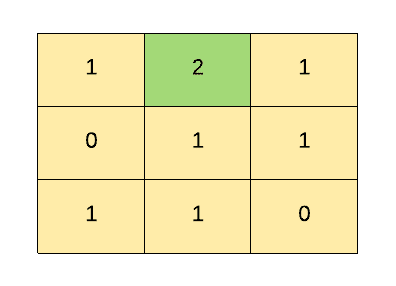The diagram above demonstrates how large the squares may be built by utilising each element as the top-left element.

Let us now discuss the Pseudo Code for the same:-

### Pseudo Code

``````public int maximalSquare(int[][] matrix)
int[][] dp = new int[rows][cols]

for(int i=rows;i>=0;i--)
for(int j=cols;j>=0;j--)
dp[i][j]=matrix[i][j]
if(i<rows-1 && j<cols-1 && dp[i][j]!=0)
dp[i][j] = 1+ Math.min(Math.min(dp[i][j+1],dp[i+1][j]) , dp[i+1][j+1])
ans = Math.max(dp[i][j],ans)
return ans * ans``````

Now, let us have a look at the Implementation:-

### Implementation in Java

``````import java.io.*;
import java.util.*;
// Main class
public class Main {
// function to obtain the maximal square
public static int maximalSquare(int[][] arr) {
//Initialized the ans as 0 because there can be a case where the matrix can be found empty
int ans = 0;
// Calculate the number of rows in a given matrix
int row = arr.length;
// Calculate the number of columns in a given matrix
int col = arr.length;
// Initialize an array with the same size of the matrix
int[][] dp = new int[row][col];

// Traverse through the given matrix from the last element
for (int i = row - 1; i >= 0; i--) {
for (int j = col - 1; j >= 0; j--) {
// Assign every element which is being traversed to the dp[][] matrix
dp[i][j] = arr[i][j];
// Check for the cases when the element is not 1, and the pointer is not in the last row, and the last column
if (i < row - 1 && j < col - 1 && dp[i][j] != 0) {

// Find out the minimum element between the horizontal, vertical, and diagonal parts and assign it to the dp[][] matrix.
dp[i][j] = Math.min(Math.min(dp[i][j + 1], dp[i + 1][j]), dp[i + 1][j + 1]) + 1;
// Check which is the greater either the current element in the dp[][] matrix or the ans.
// Each time we find the current element is greater than the ans, we update the ans.
ans = Math.max(ans, dp[i][j]);
}
// For those elements which are present in the last row or last column
ans = Math.max(ans, dp[i][j]);
}
}
// Return the area of the maximal square
return ans * ans;
}

// main function
public static void main(String[] args) {
// Scanner class to take the input from the user
Scanner scn = new Scanner(System.in);
System.out.println("Enter the number of rows: ");
int rows = scn.nextInt();
System.out.println("Enter the number of cols: ");
int cols = scn.nextInt();

int[][] arr = new int[rows][cols];

System.out.println("Enter the elements :-> ");
for (int i = 0; i < rows; i++) {
for (int j = 0; j < cols; j++) {
arr[i][j] = scn.nextInt();
}
}
// passing the array to the maximalSquare function
System.out.println("Area of the maximal Square is: " + maximalSquare(arr));
}
}``````

Input Test case-1

Output

Input Test case-2

Output

Time Complexity: O(rows x cols), where rows and cols are the total number of rows and the columns in a matrix.

Space Complexity: O(rows x cols), as we initialized the additional matrix with the same size of the given matrix.

• Does dynamic programming reduce the time complexity of programs?
Since dynamic programming isn't an algorithm, it's just a creative way to speed up some slow algorithms with a simple modification (by consuming some space). Every algorithm that can benefit from DP can be sped up in different ways.

• What are some examples of dynamic programming in practice?
In Google maps:- To discover the shortest path between a source and a set of destinations (one by one).
In networking:- To transport data sequentially from a sender to several recipients.

• What is the difference between the dynamic programming approach and the Divide and conquer approach?
The primary distinction between divide and conquer and dynamic programming is that divide and conquer combines the solutions of the subproblems to solve the main problem. In contrast, dynamic programming uses the results of the subproblems to identify the best solution to the main problem.

## Key takeaways

To summarise, we reviewed the method for determining the maximal square in a matrix. We used dynamic programming to address our challenge, which is incredibly significant for the placement. Dynamic programming is the favourite topic for recruiters. Such questions are frequently asked in interviews. Continue to pound these questions until you land your dream job.

Don't stop here, Ninja; we have a lot more ground to cover together if we desire to secure our ideal job.

Furthermore, get yourself enlisted in our top-notch courses taught by the best faculty.

Happy Coding Ninja!